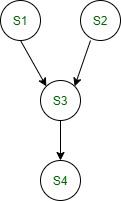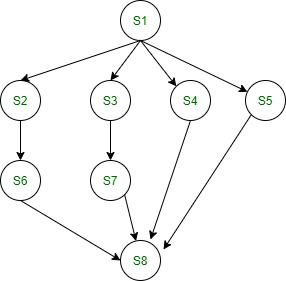Related Articles

# Concurrent Program with Precedence Graph

• Last Updated : 26 Oct, 2020

In this article, we are going to cover the Concurrent Program in the Operating System and will make a Precedence Graph for the program, and will cover the program execution for the same.

In concurrent programming, the Real concurrent program is possible on the multiprocessor system. Any two statements Si and Sj can be executed concurrently or parallel if they are following the below condition.

```Read set(Si) ∩ Write set(Sj) = ϕ
Write set(Si) ∩ Read set(Sj) = ϕ
Write set(Si) ∩ Write set(Sj) = ϕ```

In concurrent programming “Concurrent” word has a different meaning.

• They can execute concurrently or parallel.
• They do not have any dependency.
• Anyone can start first.

Example –
Let us consider the following expression –

```S1: p = q+r;
S2: s = t×u;
S3: v = w/x;
S4: y = z×a;```

Read Set and Write set for the above expression as follows –

```Read Set = {q, r, t, u, w, x, z, a}
Write Set ={p, s, v, y}```

Precedence Graph for the read and write set as follows –In concurrent programming, it will be done or return by using the following statement given below –

```par begin - par end
or
Co begin - Co end ```

Concurrent program for Precedence Graph :

Let us consider the following Precedence Graph given below –Concurrent program for the given precedence graph as follows –

```begin
S1:
par begin
begin
S2;
S6;
end
begin
S3;
S7;
end
S4;
S5;
par end
S8;
end```

Attention reader! Don’t stop learning now. Get hold of all the important CS Theory concepts for SDE interviews with the CS Theory Course at a student-friendly price and become industry ready.

My Personal Notes arrow_drop_up